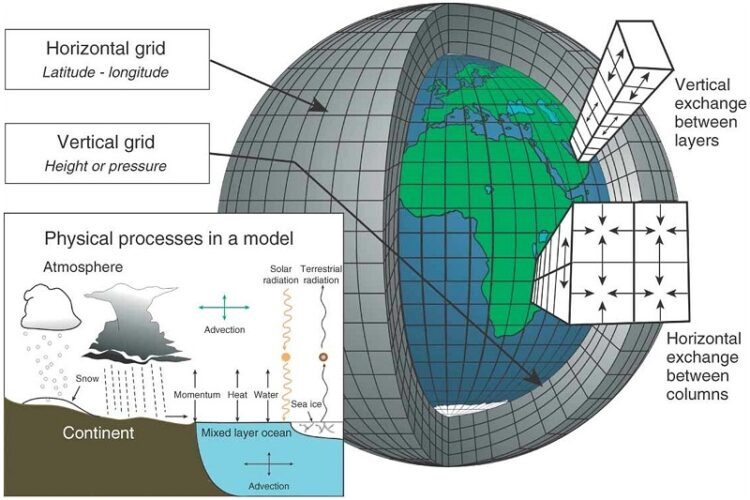# How is Numerical Modeling beneficial to the environment?Numerical modeling is a widely applied technique to simulate geological scenarios. It helps in studying rock mechanics, the thermal history of rocks, tectonic plate movements, and the Earth’s mantle. However, it is challenging to make numerical models considering all the factors affecting a system. This is because complex systems are impossible to model using a single set of equations.

Improved Decision-Making

Numerical modeling can be a powerful tool in helping decision-makers to understand and mitigate risk. It allows them to forecast the effects of specific decisions on a system at a fraction of the time and expense it would take to develop a physical model. For example, the simulation of a dam’s potential failure during a flood can be used to assess whether it is likely to breach before it does. This can save dam owners time and money while ensuring they meet regulatory requirements. However, it is essential to note that predicting the future accurately is challenging due to the complexities and conceptualization errors present in a typical physical problem. To improve this process, we have developed a workflow incorporating uncertainty quantification early before undertaking history matching. This enables modeling teams to make more informed model design and deployment decisions that will improve their models’ performance.

Reduced Errors

Engineers and scientists use numerical modeling to solve various engineering problems and check slope stability. They may be exploring new conditions or trying to validate the design of a system. A numerical model generally breaks down a problem into a discrete number of easily calculated values using robust assumptions, which are then solved by a computer. Methods include the Taylor series, Newton’s strategy, and function convergence or divergence. Errors in modeling can arise from several sources, including rounding errors and data uncertainty. This is why it is essential to minimize these errors when performing a numerical model. This allows engineers to solve many equations quickly and accurately, which is especially useful for solving initial value or long-term forecasting problems.

Reduced Costs

Whether for engineering analysis, a product, or a process, numerical modeling can help reduce costs in the long run. It can provide information that would otherwise require expensive experiments, and often, this is the only way to gain the necessary knowledge. The first step is to determine the objective of the modeling process and develop a scope that aligns with it. This first step helps ensure the project is on track and meets all stakeholder expectations. Another critical step is to create a calibrated numerical model that can be used for prediction and verification purposes. This can be done using machine learning techniques.

Increased Efficiency

Engineering has long relied on theory and experiments to obtain needed information, but the rise of computer technology has made it possible to create engaging tools to analyze problems. Numerical modeling adds another approach to the mix that can provide information not possible through other methods. There are two basic approaches to mathematical modeling. The first involves a physical model based on the fundamental physics of the system. When a model is constructed, it must be able to represent the physics of the system at the level of detail that is required by the problem. For example, this can include network flow models or heat exchanger heat transfer correlations. The second approach is a numerical model requiring high-powered computers to solve complex mathematical equations. This can consist of various formulations, but the key is finding an algorithm that will provide a reliable approximation of the exact solution.